# Linked List 1 List n A list refers

• Slides: 39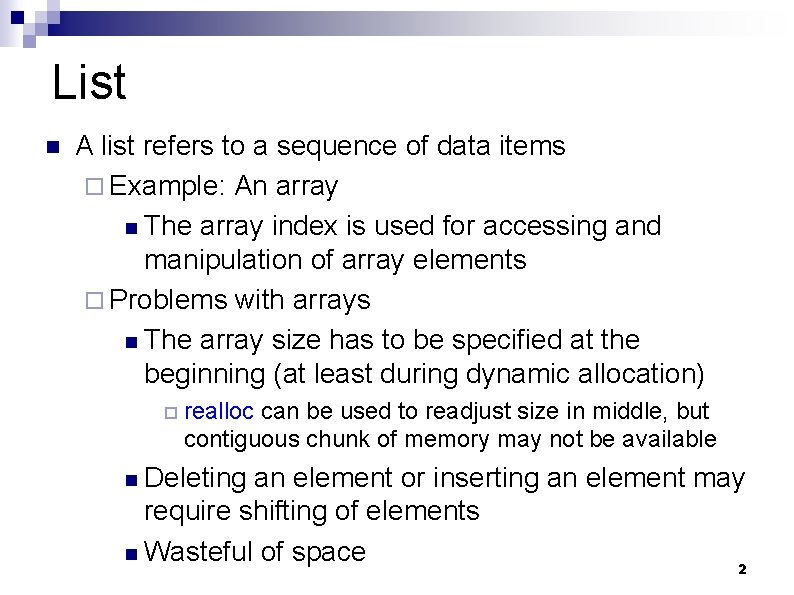List n A list refers to a sequence of data items ¨ Example: An array n The array index is used for accessing and manipulation of array elements ¨ Problems with arrays n The array size has to be specified at the beginning (at least during dynamic allocation) ¨ realloc can be used to readjust size in middle, but contiguous chunk of memory may not be available Deleting an element or inserting an element may require shifting of elements n Wasteful of space 2 n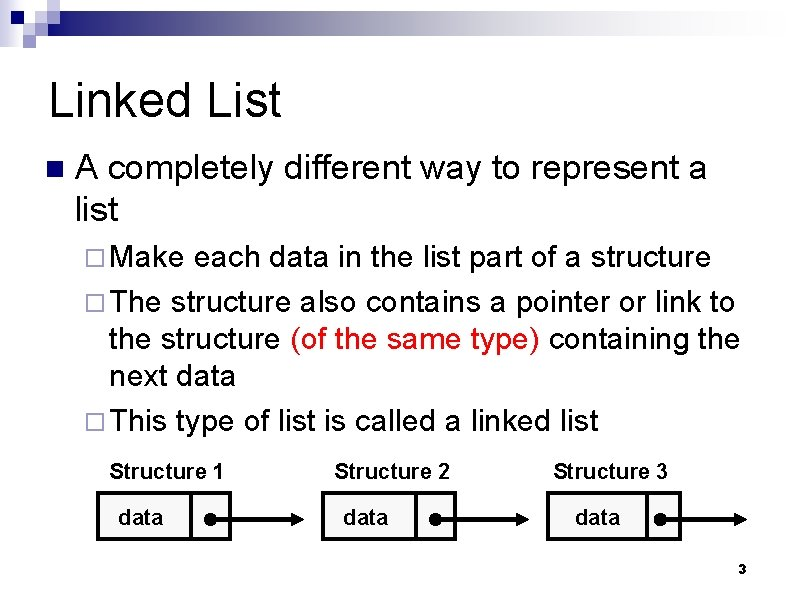Linked List n A completely different way to represent a list ¨ Make each data in the list part of a structure ¨ The structure also contains a pointer or link to the structure (of the same type) containing the next data ¨ This type of list is called a linked list Structure 1 data Structure 2 data Structure 3 data 3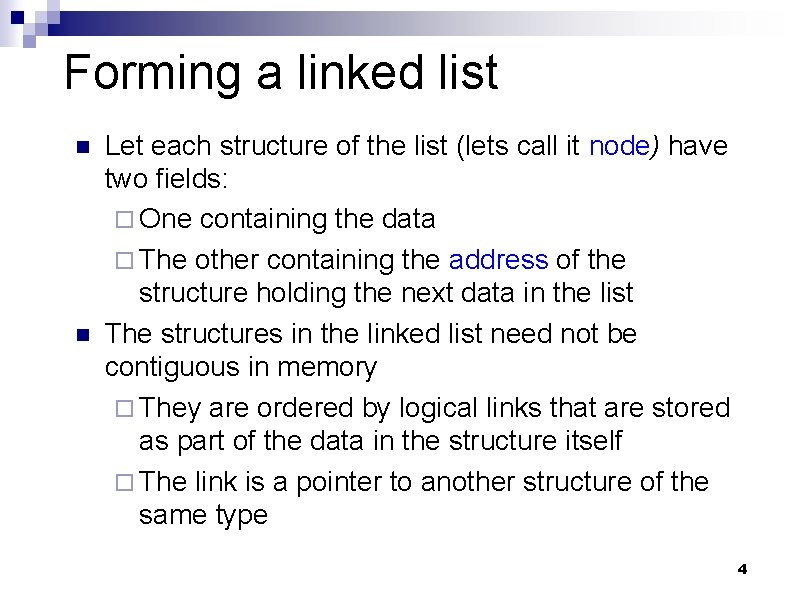Forming a linked list n n Let each structure of the list (lets call it node) have two fields: ¨ One containing the data ¨ The other containing the address of the structure holding the next data in the list The structures in the linked list need not be contiguous in memory ¨ They are ordered by logical links that are stored as part of the data in the structure itself ¨ The link is a pointer to another structure of the same type 4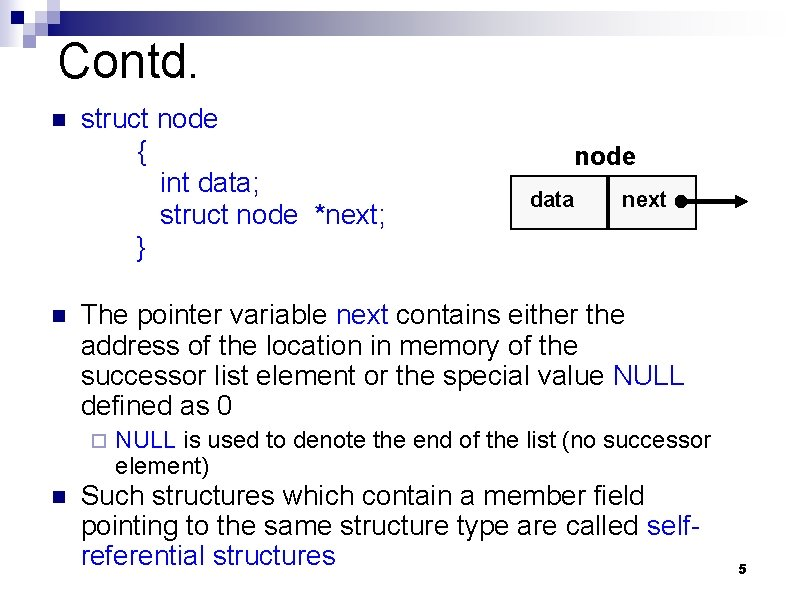Contd. n n struct node { int data; struct node *next; } data next The pointer variable next contains either the address of the location in memory of the successor list element or the special value NULL defined as 0 ¨ n node NULL is used to denote the end of the list (no successor element) Such structures which contain a member field pointing to the same structure type are called selfreferential structures 5Example: nodes of the list struct node a, b, c; a. data = 1; b. data = 2; c. data = 3; a. next = b. next = c. next = NULL; a b 1 NULL data next c 2 NULL data next 3 NULL data next 6Chaining these together a. next = &b; b. next = &c; a b 1 data next c 2 data next 3 NULL data next What are the values of : • a. next->data 7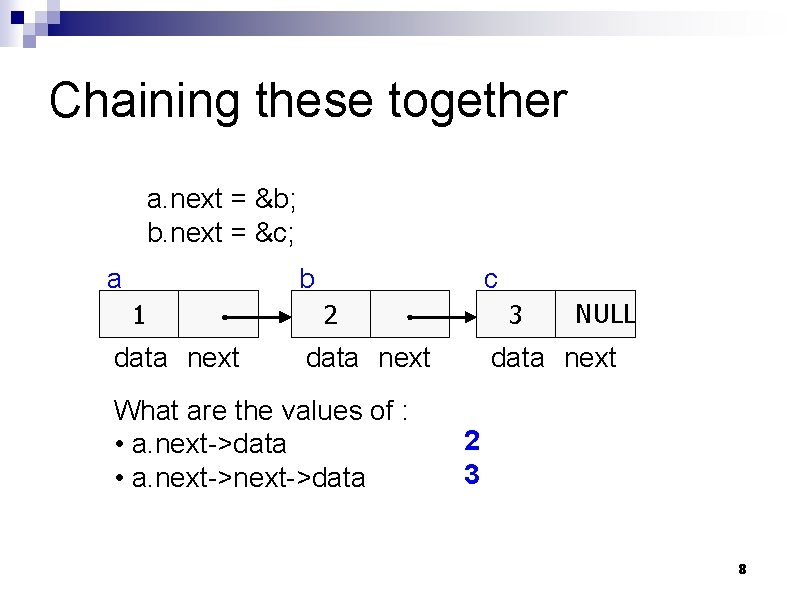Chaining these together a. next = &b; b. next = &c; a b 1 data next c 2 3 data next What are the values of : • a. next->data NULL data next 2 3 8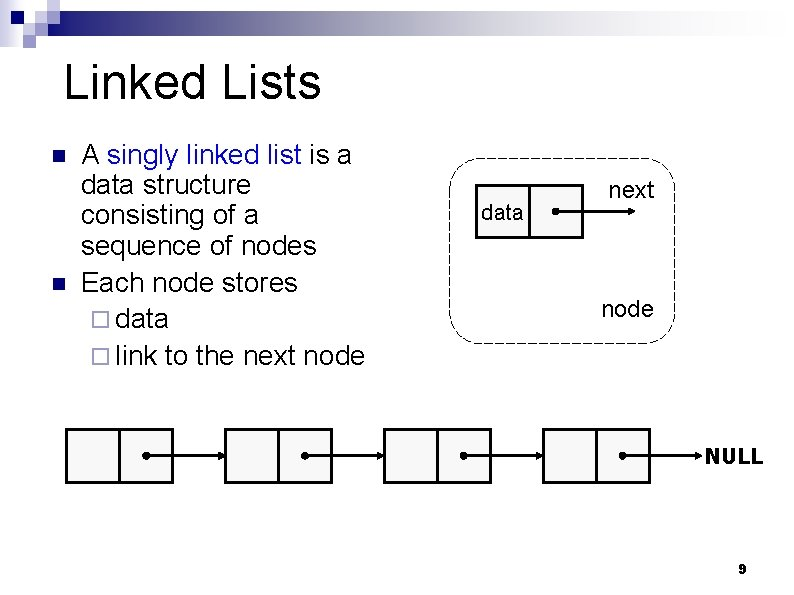Linked Lists n n A singly linked list is a data structure consisting of a sequence of nodes Each node stores ¨ data ¨ link to the next node data next node NULL 9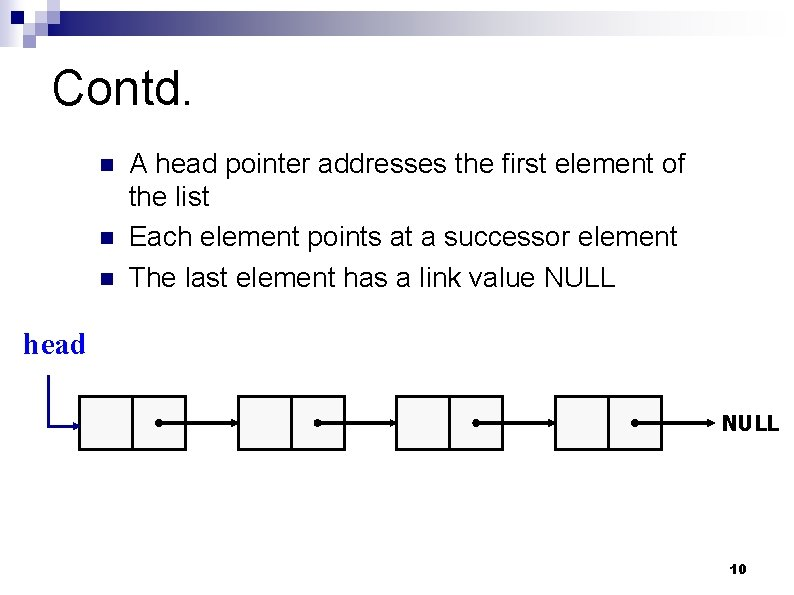Contd. n n n A head pointer addresses the first element of the list Each element points at a successor element The last element has a link value NULL head NULL 10Contd. n In general, a node of the linked list may be represented as Name of the type of nodes struct node_name { Data items in each type member 1; element of the list type member 2; ……… Link to the next struct node_name *next; element in the list }; 11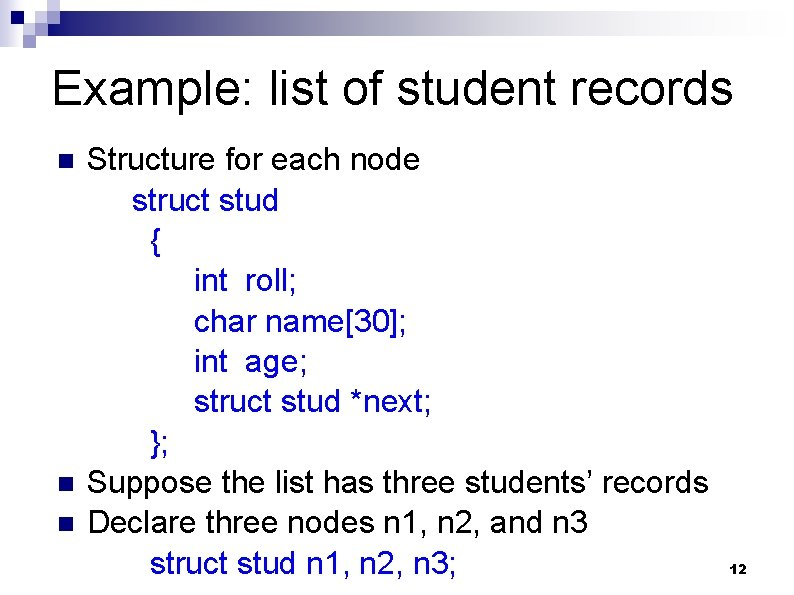Example: list of student records n n n Structure for each node struct stud { int roll; char name; int age; struct stud *next; }; Suppose the list has three students’ records Declare three nodes n 1, n 2, and n 3 struct stud n 1, n 2, n 3; 12Contd. n n Create the links between the nodes n 1. next = &n 2 ; n 2. next = &n 3 ; n 3. next = NULL ; /* No more nodes follow */ The final list looks like roll name age next n 1 n 2 n 3 13Code for the Example #include <stdio. h> struct stud { int roll; char name; int age; struct stud *next; }; int main() { struct stud scanf (“%d %s n 1, n 2, n 3; *p; %d”, &n 1. roll, n 1. name, &n 1. age); %d”, &n 2. roll, n 2. name, &n 2. age); %d”, &n 3. roll, n 3. name, &n 3. age); 14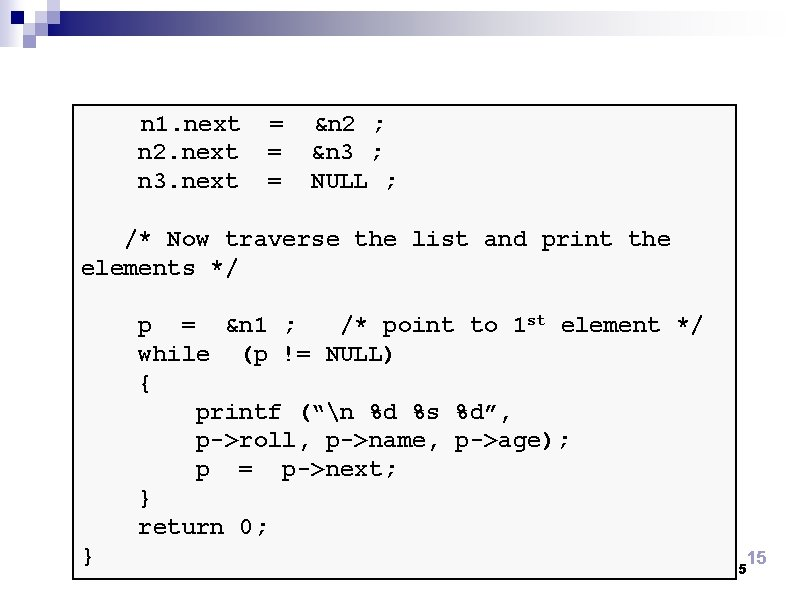n 1. next n 2. next n 3. next = = = &n 2 ; &n 3 ; NULL ; /* Now traverse the list and print the elements */ p = &n 1 ; /* point to 1 st element */ while (p != NULL) { printf (“n %d %s %d”, p->roll, p->name, p->age); p = p->next; } return 0; } 15 15Alternative Way n Instead of statically declaring the structures n 1, n 2, n 3, ¨ Dynamically allocate space for the nodes ¨ Use malloc individually for every node allocated n n This is the usual way to work with linked lists, as number of elements in the list is usually not known in advance (if known, we could have used arrays) See examples next 16 16Example of dynamic node allocation Storing a set of elements = {15, 18, 12, 7} 15 18 struct node { int data ; struct node * next ; }; struct node *p, *q; 12 7 NULL data int next node * 17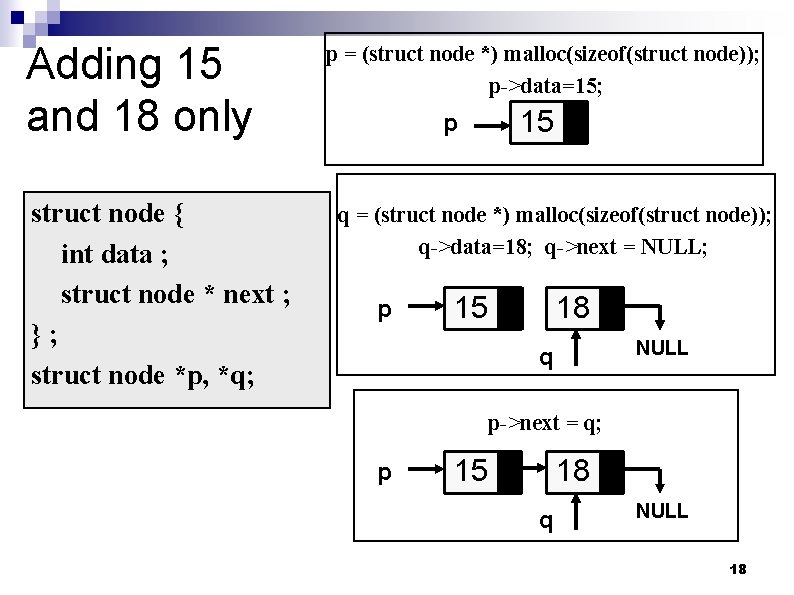Adding 15 and 18 only struct node { int data ; struct node * next ; }; struct node *p, *q; p = (struct node *) malloc(sizeof(struct node)); p->data=15; p 15 q = (struct node *) malloc(sizeof(struct node)); q->data=18; q->next = NULL; p 15 18 NULL q p->next = q; p 15 18 q NULL 18Traversing the elements added struct node { int data; struct node * next; }; int main() { struct node *p, *q, *r; p = (struct node *) malloc(sizeof(struct node)); : r=p; while(r!=NULL){ printf("Data = %d n", r->data); r=r->next; } return 0; } Output Data = 15 Data = 18 We could have done anything else other than printing as we traverse each element, like searching for example. Just like traversing an array. 19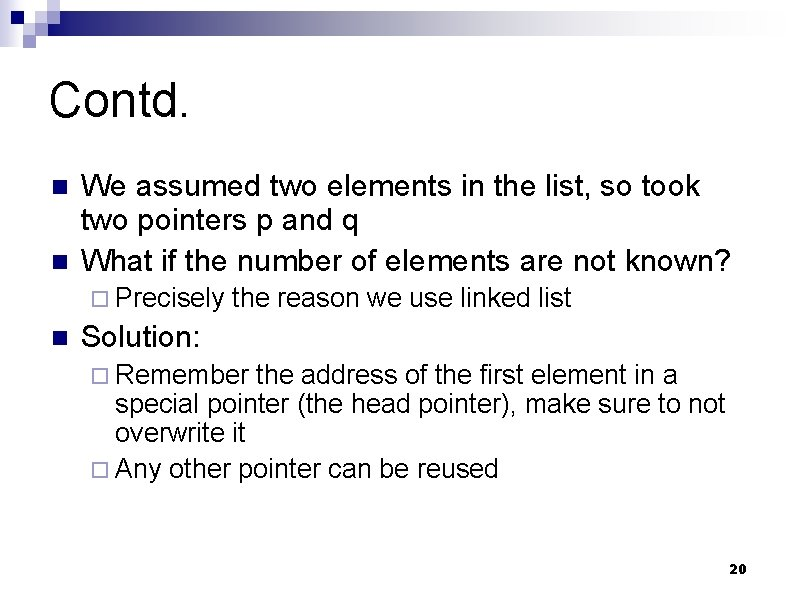Contd. n n We assumed two elements in the list, so took two pointers p and q What if the number of elements are not known? ¨ Precisely n the reason we use linked list Solution: ¨ Remember the address of the first element in a special pointer (the head pointer), make sure to not overwrite it ¨ Any other pointer can be reused 20Example: adding n elements read from keyboard int main() { int n, i; struct node *head = NULL, *prev; scanf(“%d”, &n); for (i = 0; i < n; ++i) { p = (struct node *) malloc(sizeof(struct node)); scanf(“%d”, &p->data); p->next = NULL; if (head == NULL) head = p; else prev->next = p; prev = p; } return 0; } head changes only once, when the first element is added prev remembers the pointer to the last element added, p is linked to its next field p and prev are reused as many times as needed 21Example: printing an arbitrary sized list int main() { int n, i; struct node *head = NULL, *p; : p = head; while (p != NULL) { printf(“%d “, p->data); p = p->next; } return 0; } Assumed that the list is already created and head points to the first element in the list p is reused to point to the elements in the list (initially, to the first element) When p points to the last element, p>next = NULL, so the loop terminates after this iteration 22Important to remember n Store the address of the first element added in a separate pointer (head in our examples), and make sure not to change it ¨ If you lose the start pointer, you cannot access any element in the list, as elements are only accessible from the next pointers in the previous element ¨ In the print example, we could have reused head, (head=head->next instead of p=p->next) as we do not need to remember the start pointer after printing the list, but this is considered bad practice, so we used a separate temporary pointer p 23Function to print a list The pointer to the start of the list (head pointer) is passed as parameter void display (struct node *r) { struct node *p = r; printf(“List = {”); while(p != NULL) { printf("%d, ", p->data); p = p->next; } printf(“}n”); } 24Common Operations on Linked Lists n n Creating a linked list (already seen) Printing a linked list (already seen) Search for an element in a linked list (can be easily done by traversing) Inserting an element in a linked list ¨ Insert at front of list ¨ Insert at end of list ¨ Insert in sorted order n Delete an element from a linked list 25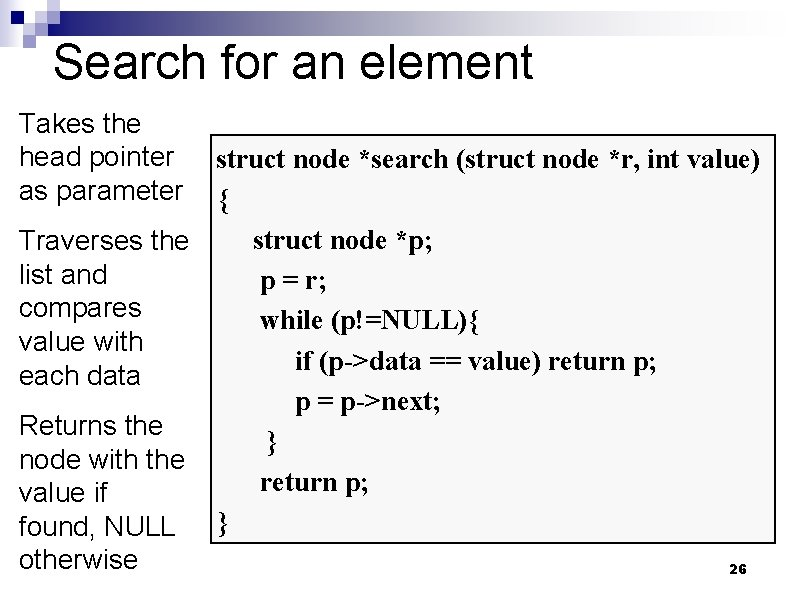Search for an element Takes the head pointer as parameter struct node *search (struct node *r, int value) { struct node *p; Traverses the list and p = r; compares while (p!=NULL){ value with if (p->data == value) return p; each data p = p->next; Returns the } node with the return p; value if found, NULL } otherwise 26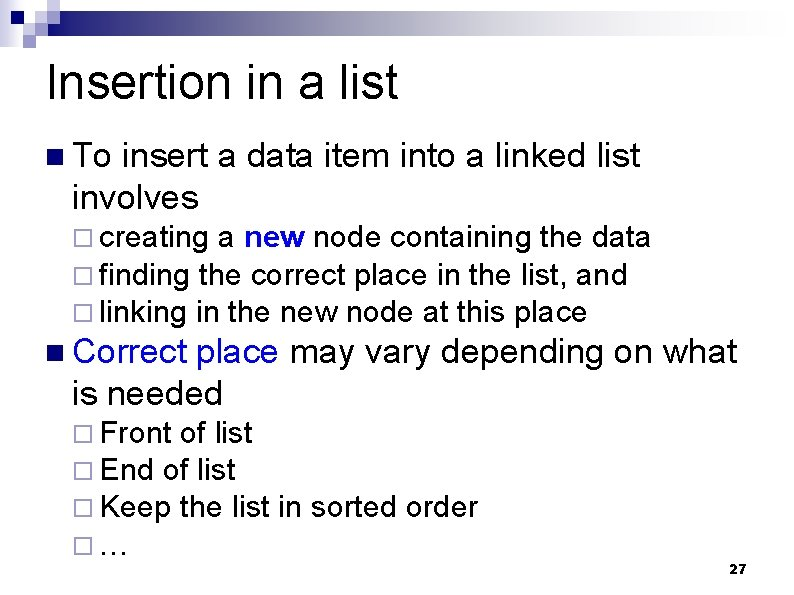Insertion in a list n To insert a data item into a linked list involves ¨ creating a new node containing the data ¨ finding the correct place in the list, and ¨ linking in the new node at this place n Correct place may vary depending on what is needed ¨ Front of list ¨ End of list ¨ Keep the list ¨… in sorted order 27Takes the head pointer and the value to be inserted (NULL if list is empty) Inserts the value as the first element of the list Returns the new head pointer value Insert in front of list struct node *insert(struct node *r, int value) { struct node *p; p = (struct node *) malloc(sizeof(struct node)); p->data = value; p ->next = r; return p; } 28Contd. 18 … 3 r 15 18 p 3 … r 29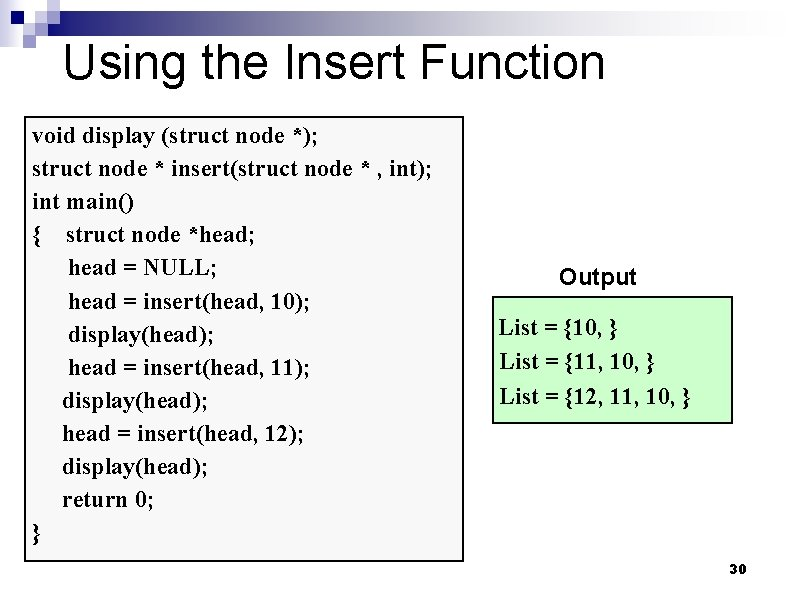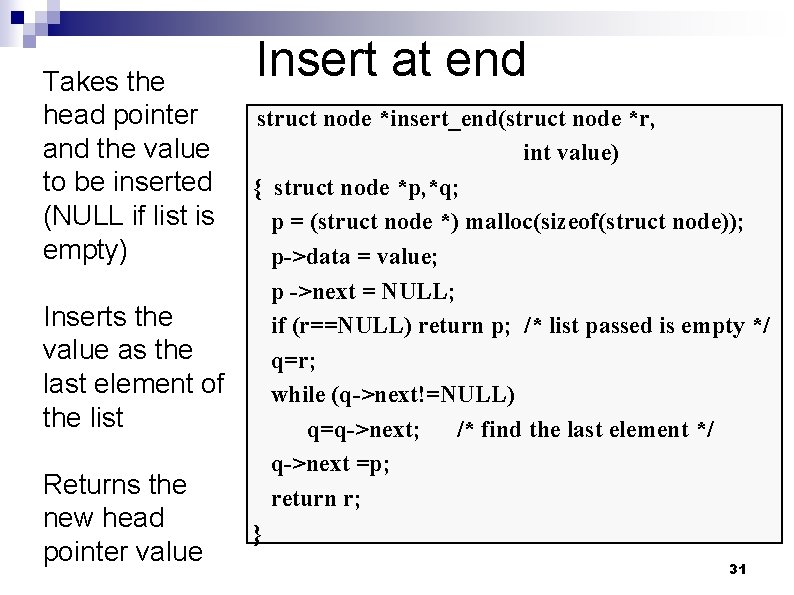Takes the head pointer and the value to be inserted (NULL if list is empty) Inserts the value as the last element of the list Returns the new head pointer value Insert at end struct node *insert_end(struct node *r, int value) { struct node *p, *q; p = (struct node *) malloc(sizeof(struct node)); p->data = value; p ->next = NULL; if (r==NULL) return p; /* list passed is empty */ q=r; while (q->next!=NULL) q=q->next; /* find the last element */ q->next =p; return r; } 31Contd. 11 4 … r NULL q p 11 4 … r 15 NULL q NULL p 11 r … 4 15 q NULL 32Using the Insert at End Function void display (struct node *); struct node * insert(struct node * , int); struct node * insert_end(struct node * , int); int main() { struct node *start; start = NULL; start = insert_end(start, 10); display(start); start = insert_end(start, 11); display(start); start = insert_end(start, 12); display(start); return 0; } Output List = {10, } List = {10, 11, 12, } 33Insertion in Ascending Order first prev 3 5 new n n ptr 8 12 - 7 Create new node for the 7 Find correct place – when ptr finds the 8 (7 < 8) Link in new node with previous (even if last) and ptr nodes Also check insertion before first node! 34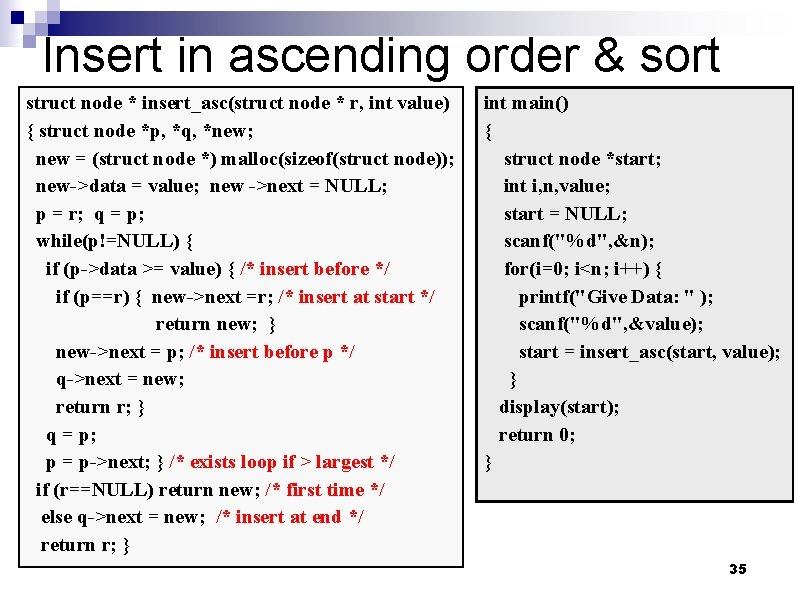Insert in ascending order & sort struct node * insert_asc(struct node * r, int value) { struct node *p, *q, *new; new = (struct node *) malloc(sizeof(struct node)); new->data = value; new ->next = NULL; p = r; q = p; while(p!=NULL) { if (p->data >= value) { /* insert before */ if (p==r) { new->next =r; /* insert at start */ return new; } new->next = p; /* insert before p */ q->next = new; return r; } q = p; p = p->next; } /* exists loop if > largest */ if (r==NULL) return new; /* first time */ else q->next = new; /* insert at end */ return r; } int main() { struct node *start; int i, n, value; start = NULL; scanf("%d", &n); for(i=0; i<n; i++) { printf("Give Data: " ); scanf("%d", &value); start = insert_asc(start, value); } display(start); return 0; } 35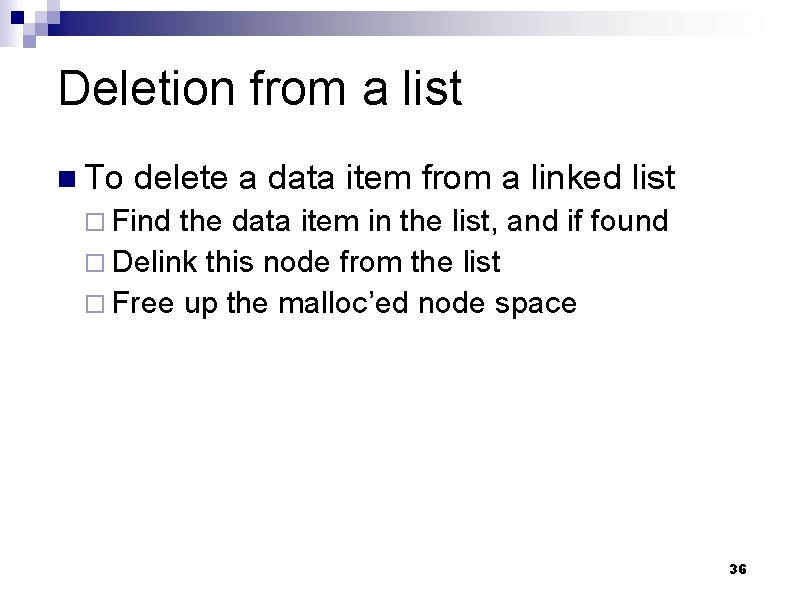Deletion from a list n To delete a data item from a linked list ¨ Find the data item in the list, and if found ¨ Delink this node from the list ¨ Free up the malloc’ed node space 36Example of Deletion first 3 n n prev 5 ptr 8 12 - When ptr finds the item to be deleted, e. g. 8, we need the previous node to make the link to the next one after ptr (i. e. ptr -> next) Also check whether first node is to be deleted 37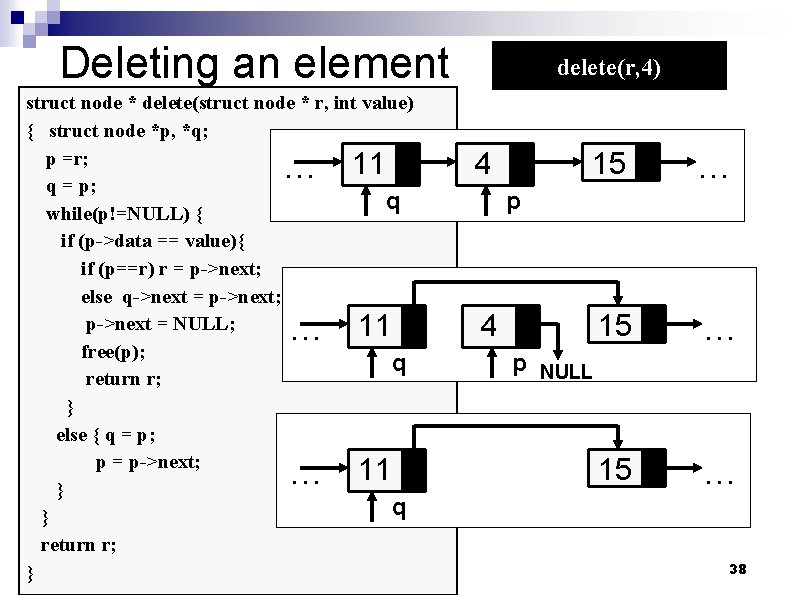Deleting an element struct node * delete(struct node * r, int value) { struct node *p, *q; p =r; 11 q = p; q while(p!=NULL) { if (p->data == value){ if (p==r) r = p->next; else q->next = p->next; p->next = NULL; 11 free(p); q return r; } else { q = p; p = p->next; 11 } q } return r; } … … … delete(r, 4) 4 15 … p 4 p NULL 38Exercises Print a list backwards (try a recursive print) n Count the number of elements in a list (both using and not using recursion) n Concatenate two lists n Reverse a list n 39